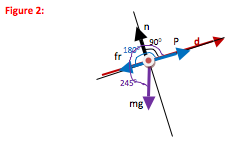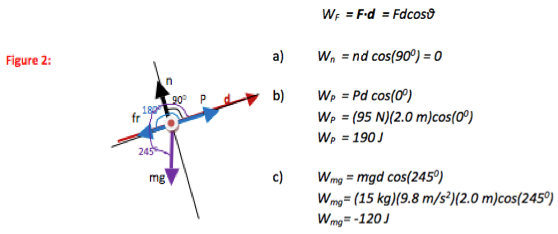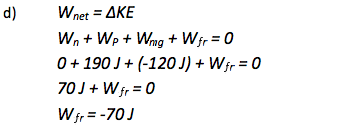# University of Wisconsin Green Bay

You push a 15 kg box of books 2.0 m up a 25o incline into the back of a moving van. The box moves at a constant velocity if you push it with a force of 95 N. Find a) the work done by normal force on the box, b) the work done by your push on the box, c) the work done by gravity on the box, and d) the work done by friction on the box. (Assume your push is parallel to the incline.)

• In this problem, you are given information about forces on an object and the distance it moves, and you are asked for work. Force and work are closely related through the definition of work. Parts a), b), and c) are definition problems.

You are not directly told the magnitude of the frictional force. Therefore, part d) is not a definition problem. However, you do know the motion of the box. Work and motion are related through the Work-Energy Theorem in the same way that force and motion are related through Newton’s Second Law. If you don’t recognize that there will be a Work-Energy Theorem component to this problem now, that is fine. It will become apparent when you get to part d) of the problem.

•Because the definition of work depends on the angle between force and displacement, it is helpful to draw a picture even though this is a definition problem. The picture needs to show that angle for each force in question. To show the angle, begin in the direction of displacement and rotate counter-clockwise to the force. It is fine to draw a separate picture for each force, rather than color-coding the angles as done here.

•In equation form, the definition of the work done by force F is

WF = F∙d = Fdcosθ

This is the only relation that you need for parts (a-c) of this problem.

In part d), you are not given information about the size of the frictional force. You can find it using Newton’s Second Law and then use the definition of work once again. However, whenever you are asked about work it is easier to use the Work-Energy Theorem in place of Newton’s Second Law if possible. In equation form, the Work-Energy Theorem is

Wnet = ΔKE

• Step 1If you did not recognize that you would need to use the Work-Energy Theorem to solve part d) of this problem earlier, you would see it now. You do not know the size of the frictional force and so cannot just plug it into the definition equation. Continue to Step 2 to solve part d) using the Work-Energy Theorem.

Step 2Part d) of this problem asked for the work done on the box by the frictional force. No further mathematical solution is necessary.

• In this problem, we were asked to find the work done on a box by a variety of forces. When you know the magnitude of a force, the work is does is given by: